# Visualizing Rauzy fractals via finite directed graphs

Date started: September 2019
Leads: Dina Buric

###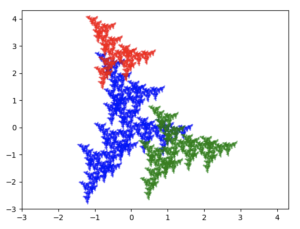Abstract

The purpose of the project was to visualize the possible images of a projection mapping. The necessary data consisted of a labeled finite directed graph coming from a volume preserving hyperbolic matrix. Projecting all possible bi-infinite paths of the graph onto the contracting eigenspace of the matrix produces fractal like sets. It was a struggle to visualize these sets on my own and this is where technology helped me out. I used the graph from the Tribonacci substitution as an example to write a program that produced pictures of the fractal like sets from random labelings on the graph. The code for the sets was written in Python and is available on my github page. The types of possible sets have been surprising and I am currently trying to understand the correspondence between the labels and the sets produced. Many more examples can be found on the Twitter Bot, updated daily (most of the time) with links describing the mathematics behind the projection mapping.

### Media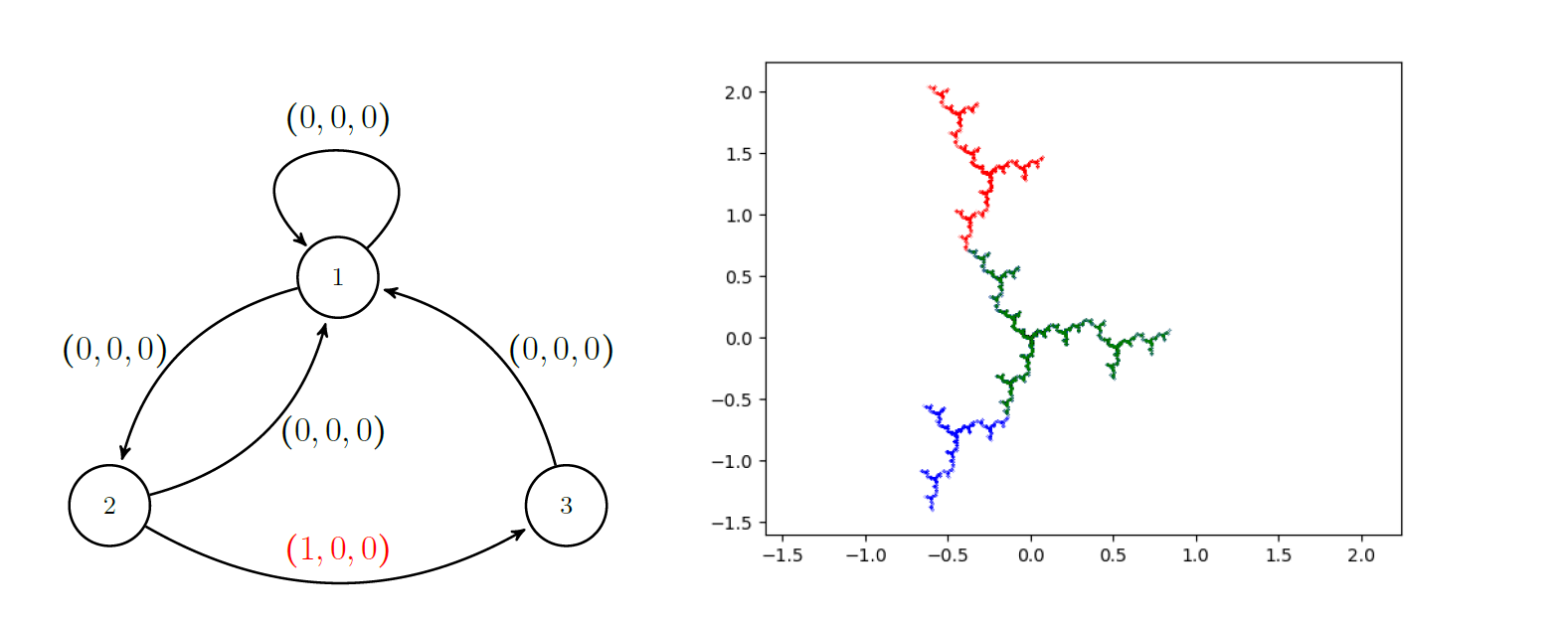The Tribonacci graph with only one non-zero edge label and the corresponding fractal like set, a nice looking dendrite.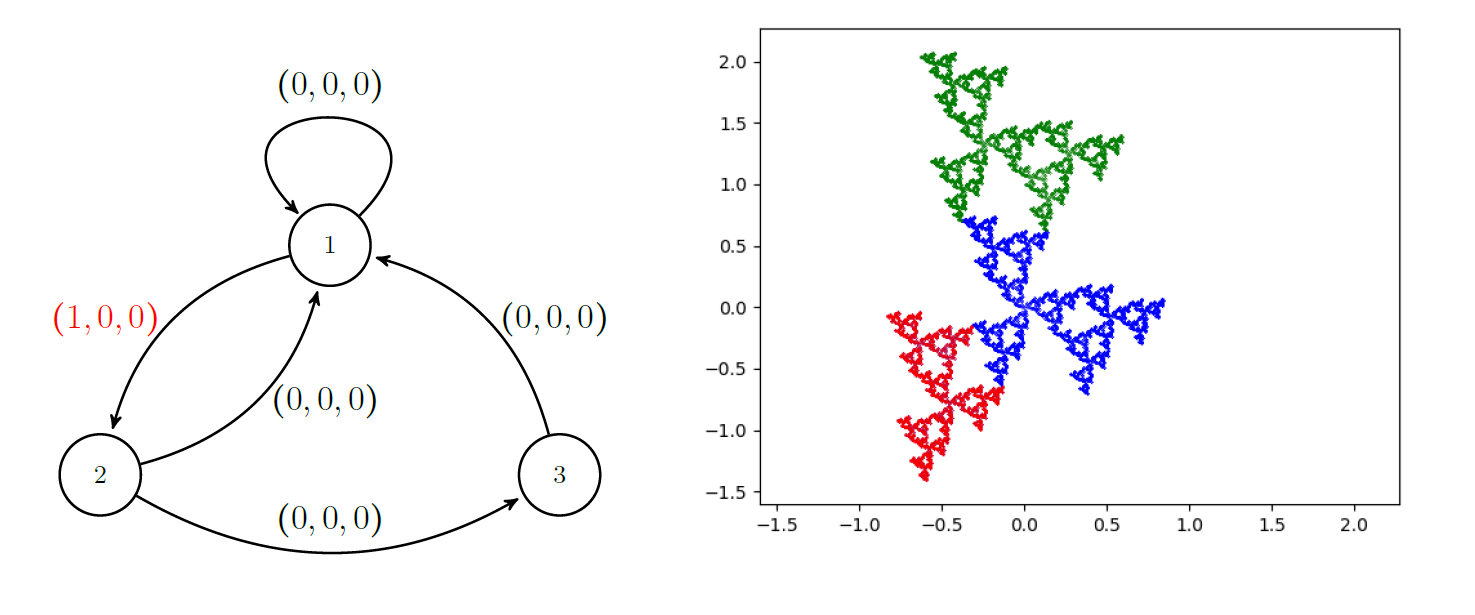Another Tribonacci graph with only one non-zero edge label but with a different fractal like set.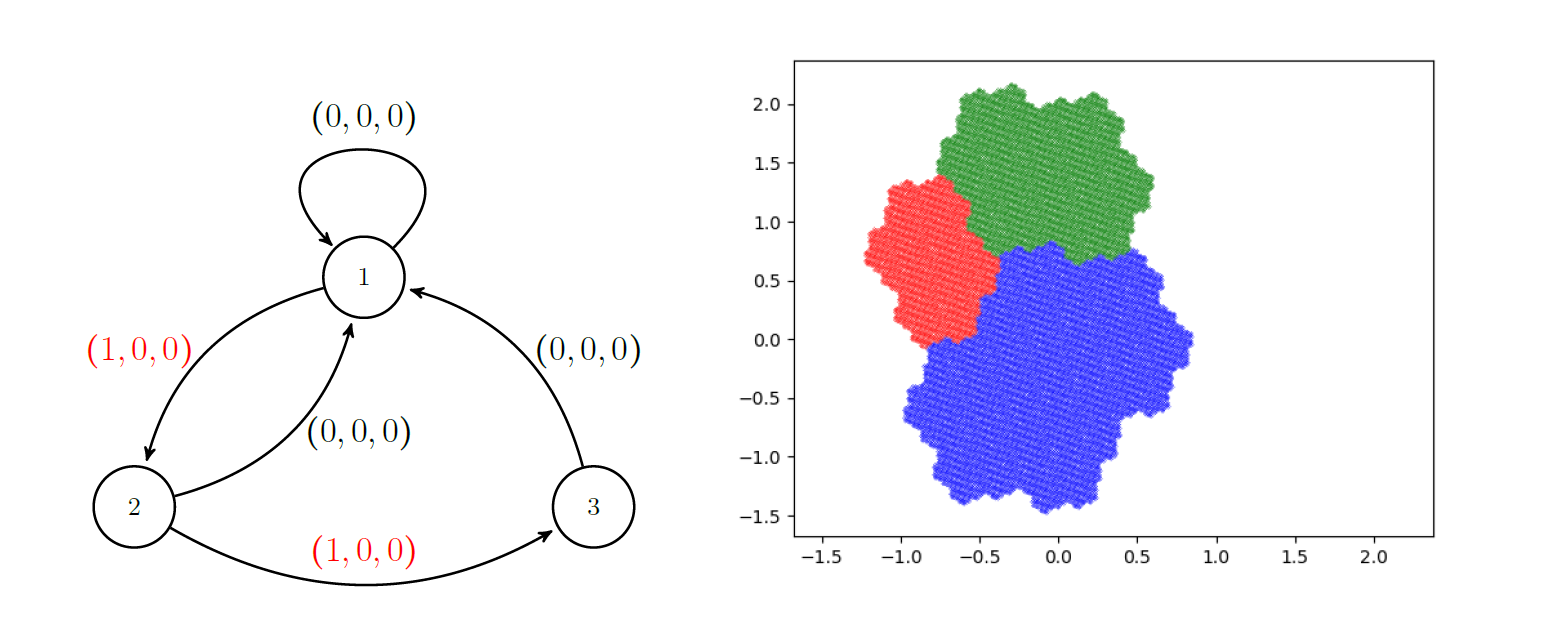Combining the two previous examples we get a graph with two non-zero labels and miraculously, a regular set with fractal boundary, called the Rauzy fractal.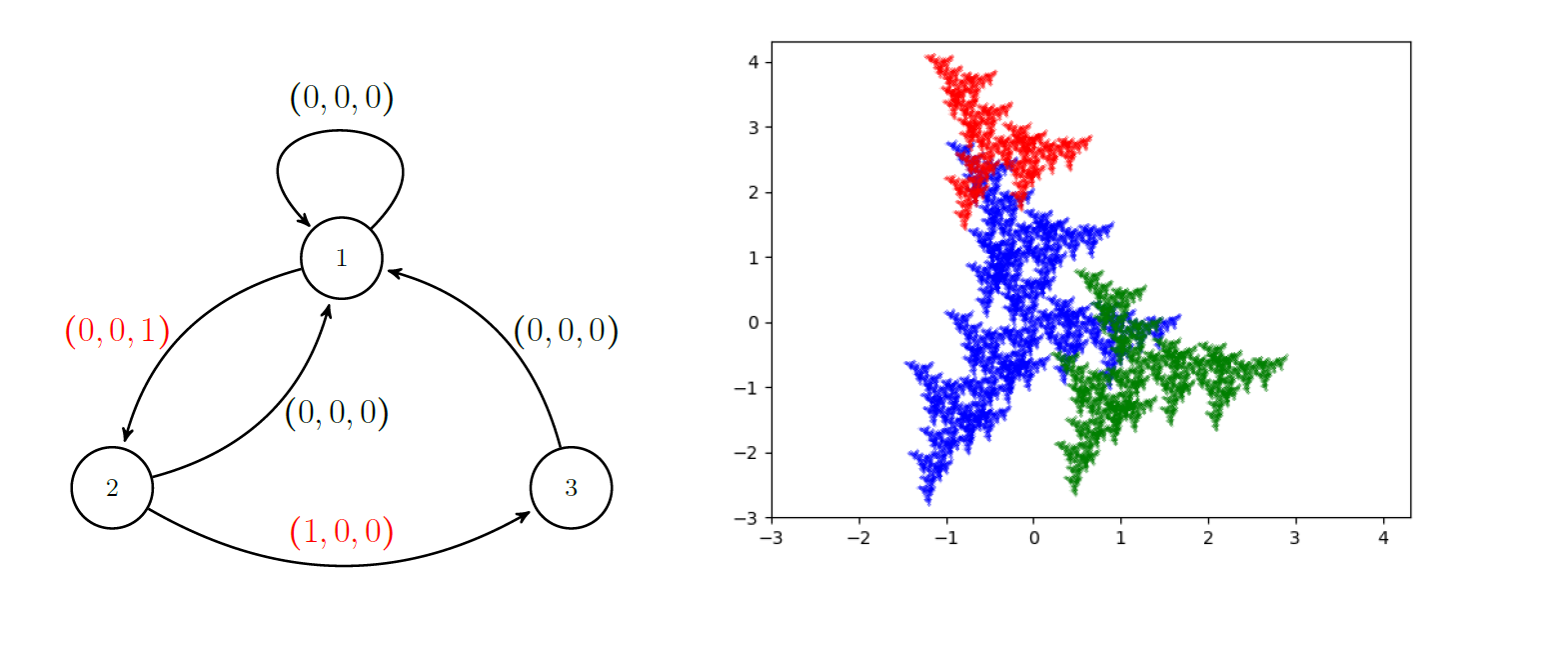Interestingly enough, slightly changing one of the labels from example three gives a completely different fractal like set.

### References

• N. Pytheas Fogg: Substitutions in Dynamics, Arithmetics and Combinatorics
• Pierre Arnoux and Shunji Ito: Pisot Substitutions and Rauzy Fractals